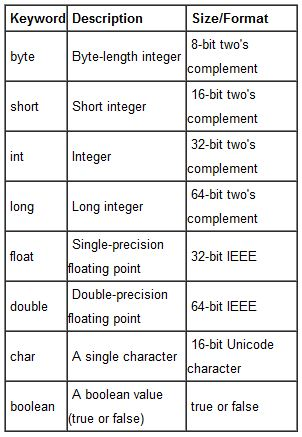## Thursday, September 22, 2011

### Learn Core JAVA in Easy Steps [Page 3]

JAVA Data Types :-
(1) Primitive :
1. Integral
2. Boolean
3. Floating
(2) Reference :
1. Object
2. String

• byte: The `byte` data type is an 8-bit signed two's complement integer. It has a minimum value of -128 and a maximum value of 127 (inclusive). The `byte` data type can be useful for saving memory in large arrays, where the memory savings actually matters. They can also be used in place of `int` where their limits help to clarify your code; the fact that a variable's range is limited can serve as a form of documentation.
• short: The `short` data type is a 16-bit signed two's complement integer. It has a minimum value of -32,768 and a maximum value of 32,767 (inclusive). As with `byte`, the same guidelines apply: you can use a `short` to save memory in large arrays, in situations where the memory savings actually matters.
• int: The `int` data type is a 32-bit signed two's complement integer. It has a minimum value of -2,147,483,648 and a maximum value of 2,147,483,647 (inclusive). For integral values, this data type is generally the default choice unless there is a reason (like the above) to choose something else. This data type will most likely be large enough for the numbers your program will use, but if you need a wider range of values, use `long` instead.
• long: The `long` data type is a 64-bit signed two's complement integer. It has a minimum value of -9,223,372,036,854,775,808 and a maximum value of 9,223,372,036,854,775,807 (inclusive). Use this data type when you need a range of values wider than those provided by `int`.

• float: The `float` data type is a single-precision 32-bit IEEE 754 floating point. Its range of values is beyond the scope of this discussion, but is specified in section 4.2.3of the Java Language Specification. As with the recommendations for `byte` and `short`, use a `float` (instead of `double`) if you need to save memory in large arrays of floating point numbers. This data type should never be used for precise values, such as currency. For that, you will need to use the java.math.BigDecimal class instead.Numbers and Strings covers `BigDecimal` and other useful classes provided by the Java platform.
• double: The `double` data type is a double-precision 64-bit IEEE 754 floating point. Its range of values is beyond the scope of this discussion, but is specified in section4.2.3 of the Java Language Specification. For decimal values, this data type is generally the default choice. As mentioned above, this data type should never be used for precise values, such as currency.
• boolean: The `boolean` data type has only two possible values: `true` and `false`. Use this data type for simple flags that track true/false conditions. This data type represents one bit of information, but its "size" isn't something that's precisely defined.
• char: The `char` data type is a single 16-bit Unicode character. It has a minimum value of `'\u0000'` (or 0) and a maximum value of `'\uffff'` (or 65,535 inclusive).Data TypeDefault Value (for fields)
byte0
short0
int0
long0L
float0.0f
double0.0d
char'\u0000'
String (or any object)  null
booleanfalse